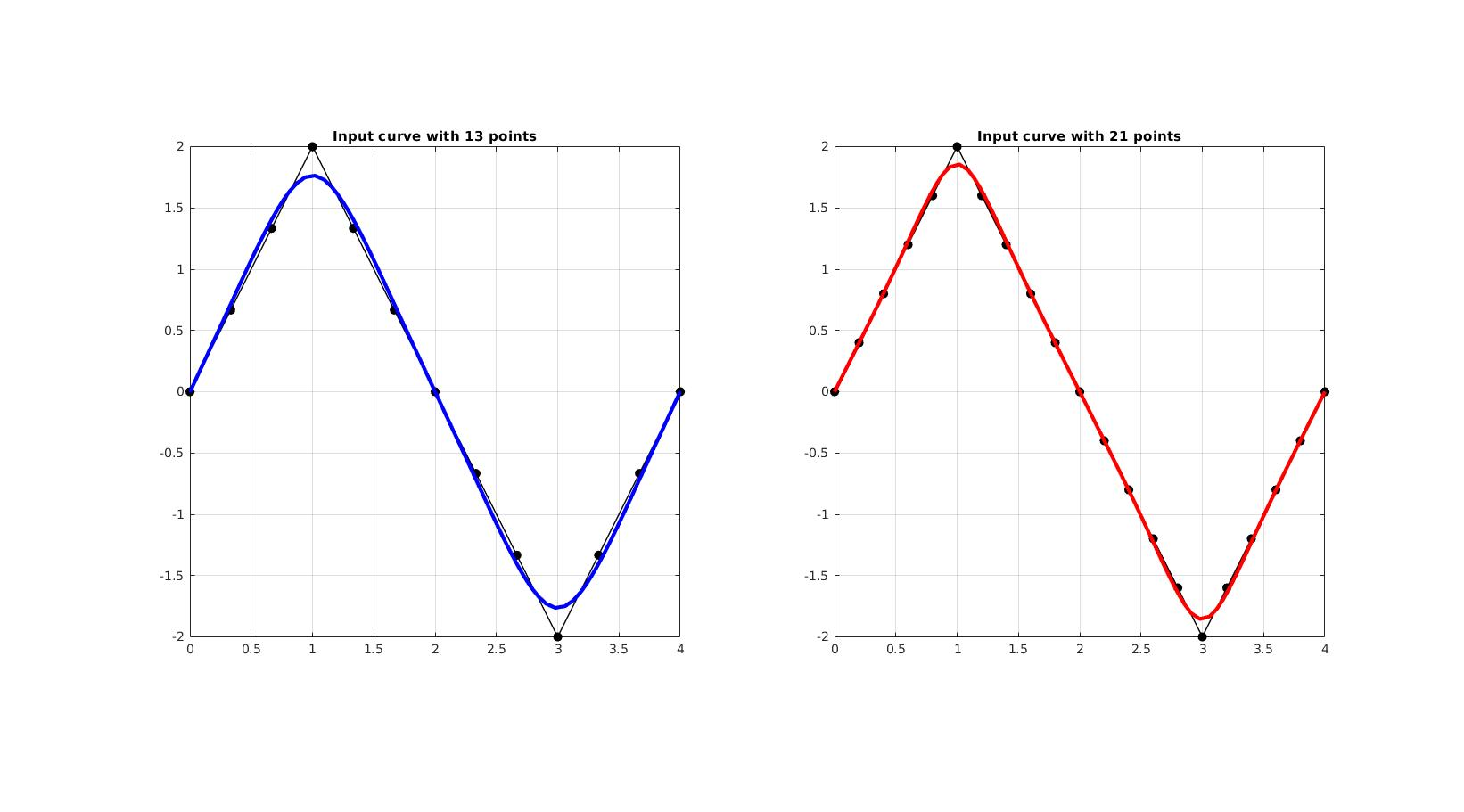# csapsPar

Below is a demonstration of the features of the csapsPar function

## Contents

```clear; close all; clc;
```

## Syntax

p=csapsPar(varargin);

## Description

This function aims to compute the cubic smoothing spline parameter p for the MATLAB csaps function. The input features the curve vertices V and the parameter pw. The smoothing parameter p is derived using:

p=1./(1+(((h.^3)/6)*f));

Where h is the point spacing (derived from V) and f is defined as:

f=(1/pw)-1;

The interesting range for p is close to 1./(1+((h.^3)/6)). The following form is used introducing the factor f: p=1./(1+(((h.^3)/6)*f)). By using f=10 we obtain p=1./(1+((h.^3)/60)) which should result in a close following of the data. If instead f=0.1 is used, leading to p=1./(1+((h.^3)/0.6)), a smoother result is obtained.

## Examples

Create saw-tooth example curve

```V=[0 0 0; 1 2 0; 2 0 0; 3 -2 0; 4 0 0];

n1=2;
n2=2*n1;
[V1]=subCurve(V,n1);
[V2]=subCurve(V,n2);
```

Computing p parameters for the cubic smoothing spline based resampling

```pw=0.5;

p1=csapsPar(V1,pw)
p2=csapsPar(V2,pw)

% Resample curves using smoothing parameters
n=100;
V1f=evenlySampleCurve(V1,n,p1,0);
V2f=evenlySampleCurve(V2,n,p2,0);
```
```p1 =

0.9354

p2 =

0.9853

```

Visualizing curves

```cFigure;
subplot(1,2,1); hold on;
title(['Input curve with ',num2str(size(V1,1)),' points'])
plotV(V1,'k.-','LineWidth',1,'MarkerSize',25);
plotV(V1f,'b-','LineWidth',3);
axis tight; axis equal; grid on; box on;

subplot(1,2,2); hold on;
title(['Input curve with ',num2str(size(V2,1)),' points'])
plotV(V2,'k.-','LineWidth',1,'MarkerSize',25);
plotV(V2f,'r-','LineWidth',3);
axis tight; axis equal; grid on; box on;

drawnow;
```GIBBON www.gibboncode.org

Kevin Mattheus Moerman, [email protected]

GIBBON footer text

GIBBON: The Geometry and Image-based Bioengineering add-On. A toolbox for image segmentation, image-based modeling, meshing, and finite element analysis.

Copyright (C) 2006-2022 Kevin Mattheus Moerman and the GIBBON contributors

This program is free software: you can redistribute it and/or modify it under the terms of the GNU General Public License as published by the Free Software Foundation, either version 3 of the License, or (at your option) any later version.

This program is distributed in the hope that it will be useful, but WITHOUT ANY WARRANTY; without even the implied warranty of MERCHANTABILITY or FITNESS FOR A PARTICULAR PURPOSE. See the GNU General Public License for more details.

You should have received a copy of the GNU General Public License along with this program. If not, see http://www.gnu.org/licenses/.